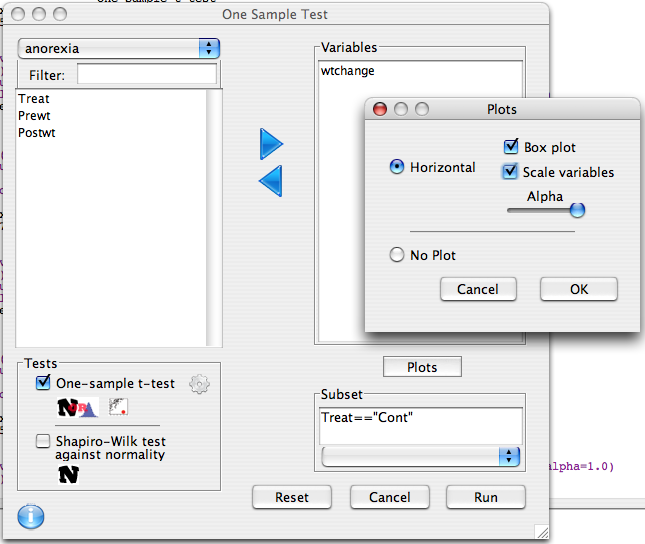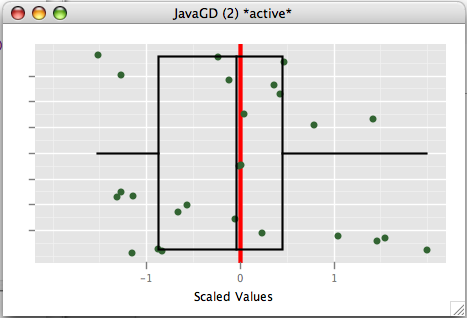## Data preparation

The anorexia data contains data on the pre and post treatment anorexia of some young female patients. We will create a new variable `wtchange` which is equal to post-weight minus pre-weight.

Treat - Factor of three levels: "Cont" (control), "CBT" (Cognitive Behavioural treatment) and "FT" (family treatment).

Prewt - Weight of patient before study period, in lbs.

Postwt- Weight of patient after study period, in lbs.

```data(anorexia)
anorexia\$wtchange <-anorexia\$Postwt - anorexia\$Prewt
```

## Dialog set-up

We will test whether the control group experienced a significant drop in weight.

1. Move wtchange to the list on the right
2. enter Treat=="Cont" into the subset field to denote that we only want to test within the control group.
3. Click plots and select box plot. Also move the alpha slider to the right so that there is no transparency in the jitter plot.## R Code

```anorexia.sub<-subset(anorexia,Treat=="Cont")
descriptive.table(anorexia.sub[c("wtchange")],func.names =c("Mean","St. Deviation","Valid N"))
one.sample.test(variables=c(wtchange),
data=anorexia.sub,
test=t.test,
alternative="two.sided")
onesample.plot(variables=c(wtchange),data=anorexia.sub,test.value=0.0,type='box',alpha=1.0)
rm(anorexia.sub)
```

## Output

```> anorexia.sub<-subset(anorexia,Treat=="Cont")
> descriptive.table(anorexia.sub[c("wtchange")],func.names =c("Mean","St. Deviation","Valid N"))
\$`strata: all cases `
Mean St. Deviation       Valid N
-0.450000      7.988705     26.000000

> one.sample.test(variables=c(wtchange),
+ data=anorexia.sub,
+ test=t.test,
+ alternative="two.sided")
One Sample t-test
mean of x 95% CI Lower 95% CI Upper          t df  p-value
wtchange     -0.45    -3.676708     2.776708 -0.2872254 25 0.776307
HA: two.sided
H0:  mean = 0
> onesample.plot(variables=c(wtchange),data=anorexia.sub,test.value=0.0,type='box',alpha=1.0)
> rm(anorexia.sub)
```

The control group lost .45 pounds on average, though this was not a significant drop (p-value = 0.776). Visually we can see from the box and jitter plot that the observations are clustered around 0.0.